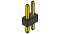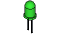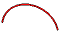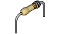﻿ products:nedx:ncir-02[.:oomlout:.]

 NCIR02
 .:8 LED Fun:..:Multiple LEDs:.(NEDX) .:Netduino Experimentation Kit:. (NEDX)

What We're Doing
We have caused one LED to blink, now it's time to up the stakes. Lets connect eight. We'll also have an opportunity to stretch the Netduino a bit by creating various lighting sequences. This circuit is also a nice setup to experiment with writing your own programs and getting a feel for how the Netduino works.

Along with controlling the LEDs we start looking into a few simple programming methods to keep your programs small.

for() loops - used when you want to run a piece of code several times.
arrays[] - used to make managing variables easier (it's a group of variables).

The Circuit
The PartsNCIR-02Breadboard Sheetx12 Pin Headerx45mm Green LEDx8Wire560 Ohm ResistorGreen-Blue-Orangex8SchematicResources
http://nedx.org/NBLS02Code (no need to type everything in just)
(and then copy the text and paste it into an empty Netduino Sketch)

//Libraries that our program uses
using System;                               //use base classes
using Microsoft.SPOT;                       //use smart personal objects technology classes
using Microsoft.SPOT.Hardware;              //use hardware related SPOT classes
using SecretLabs.NETMF.Hardware;            //use Secret Labs hardware framework
using SecretLabs.NETMF.Hardware.Netduino;   //use the Netduino specific classes

namespace NCIR02                    /// Define the namespace we are in ///
{
public class Program            /// Define any variables used in the program and subrout
//ines after Main()///
{
static OutputPort[] leds = new OutputPort;   //An array to hold the 8 pins the LE
//Ds are connected to

public static void Main()   /// The Main loop (run at power up) ///
{
/// Setup Portion of Program, runs once at startup ///
//Set each LED pin to be an output
leds = new OutputPort(Pins.GPIO_PIN_D2, false);
leds = new OutputPort(Pins.GPIO_PIN_D3, false);
leds = new OutputPort(Pins.GPIO_PIN_D4, false);
leds = new OutputPort(Pins.GPIO_PIN_D5, false);
leds = new OutputPort(Pins.GPIO_PIN_D6, false);
leds = new OutputPort(Pins.GPIO_PIN_D7, false);
leds = new OutputPort(Pins.GPIO_PIN_D8, false);
leds = new OutputPort(Pins.GPIO_PIN_D9, false);

while (true)            /// Do Forever ///
{
oneAfterAnotherNoLoop();    //Turn on each LED one by one then turn them off
//oneAfterAnotherLoop();    //This does the same as oneAfterAnotherNoLoop()
//but with much less typing
//oneOnAtATime();           //Turns each LED on one after the other
//inAndOut();               //Displays a Nightrider effect
}                       /// Close Forever Loop ///
}                           /// Close the Main() Loop ///

///
/// Will light one LED then delay for delayTime then light
/// the next LED until all LEDs are on it will then turn them off one after another.
/// This does not use a loop and is similar to the method used in NCIR01
///

static void oneAfterAnotherNoLoop()
{
int delayTime = 100;        //The time (in milliseconds) to pause between LEDs
//make smaller for quicker switching and larger for
//slower

//Turn on each LED one after another
leds.Write(true);        //Turns on LED #0 (connected to pin 2 )
leds.Write(true);        //Turns on LED #1 (connected to pin 3 )
leds.Write(true);        //Turns on LED #2 (connected to pin 4 )
leds.Write(true);        //Turns on LED #3 (connected to pin 5 )
leds.Write(true);        //Turns on LED #4 (connected to pin 6 )
leds.Write(true);        //Turns on LED #5 (connected to pin 7 )
leds.Write(true);        //Turns on LED #6 (connected to pin 8 )
leds.Write(true);        //Turns on LED #7 (connected to pin 9 )

//Turn off each LED one after another
leds.Write(false);       //Turns off LED #7 (connected to pin 9 )
leds.Write(false);       //Turns off LED #6 (connected to pin 8 )
leds.Write(false);       //Turns off LED #5 (connected to pin 7 )
leds.Write(false);       //Turns off LED #4 (connected to pin 6 )
leds.Write(false);       //Turns off LED #3 (connected to pin 5 )
leds.Write(false);       //Turns off LED #2 (connected to pin 4 )
leds.Write(false);       //Turns off LED #1 (connected to pin 3 )
leds.Write(false);       //Turns off LED #0 (connected to pin 2 )
}

///
/// Will light one LED then delay for delayTime then light
/// the next LED until all LEDs are on it will then turn them off one after another
/// This does the same as oneAfterAnotherNoLoop() but using loops which makes for a
//lot less typing.
///

static void oneAfterAnotherLoop()
{
int delayTime = 100;        //The time (in milliseconds) to pause between LEDs
//make smaller for quicker switching and larger for
//slower

//Turn each LED on one after another
for (int i = 0; i <= 7; i++)
{
leds[i].Write(true);        //Turns on LED #i each time this runs i
}                               //8 times the first time i will = 0 the final
//time i will equal 7;

//Turn each LED off one after another
for (int i = 7; i >= 0; i--)    //same as above but rather than starting at 0 an
//d counting up
{                               //we start at seven and count down
leds[i].Write(false);       //Turns off LED #i each time this runs i
Thread.Sleep(delayTime);    //gets one subtracted from it so this will repea
//t
}                               //8 times the first time i will = 7 the final
//time it will equal 0
}

///
/// Will light one LED then the next turning off all the others
///

static void oneOnAtATime()
{
int delayTime = 100;            //the time (in milliseconds) to pause between LE
//Ds
//make smaller for quicker switching and larger
//for slower
for (int i = 0; i <= 7; i++)
{
int offLED = i - 1;         //Calculate which LED was turned on last time th
//rough
if (i == 0)                 //for i = 1 to 7 this is i minus 1 (i.e. if i =
//2 we will
{                           //turn on LED 2 and off LED 1)
offLED = 7;             //however if i = 0 we don't want to turn of led
//-1 (doesn't exist)
}                           //instead we turn off LED 7, (looping around)

leds[i].Write(true);        //turn on LED #i
leds[offLED].Write(false);  //turn off the LED we turned on last time
}
}

///
/// This will turn on the two middle LEDs then the next two out
/// making an in and out look
///

static void inAndOut()
{
int delayTime = 100;            //the time (in milliseconds) to pause between LE
//Ds
//make smaller for quicker switching and larger
//for slower

//runs the LEDs out from the middle
for (int i = 0; i <= 3; i++)
{
int offLED = i - 1;     //Calculate which LED was turned on last time throug
//h
if (i == 0)
{                           //for i = 1 to 7 this is i minus 1 (i.e. if i =
//2 we will
offLED = 3;             //turn on LED 2 and off LED 1)
}                           //however if i = 0 we don't want to turn of led
//-1 (doesn't exist)
//instead we turn off LED 7, (looping around)
int onLED1 = 3 - i;         //this is the first LED to go on ie. LED #3 when
// i = 0 and LED
//#0 when i = 3
int onLED2 = 4 + i;         //this is the first LED to go on ie. LED #4 when
// i = 0 and LED
//#7 when i = 3
int offLED1 = 3 - offLED;   //turns off the LED we turned on last time
int offLED2 = 4 + offLED;   //turns off the LED we turned on last time

leds[onLED1].Write(true);
leds[onLED2].Write(true);
leds[offLED1].Write(false);
leds[offLED2].Write(false);
}

//runs the LEDs into the middle
for (int i = 3; i >= 0; i--)
{
int offLED = i + 1;         //Calculate which LED was turned on last time th
//rough
if (i == 3)
{                           //for i = 1 to 7 this is i minus 1 (i.e. if i =
//2 we will
offLED = 0;             //turn on LED 2 and off LED 1)
}                           //however if i = 0 we don't want to turn of led
//-1 (doesn't exist)
//instead we turn off LED 7, (looping around)
int onLED1 = 3 - i;         //this is the first LED to go on ie. LED #3 when
// i = 0 and LED
//#0 when i = 3
int onLED2 = 4 + i;         //this is the first LED to go on ie. LED #4 when
// i = 0 and LED
//#7 when i = 3
int offLED1 = 3 - offLED;   //turns off the LED we turned on last time
int offLED2 = 4 + offLED;   //turns off the LED we turned on last time

leds[onLED1].Write(true);
leds[onLED2].Write(true);
leds[offLED1].Write(false);
leds[offLED2].Write(false);
}
}
}                               /// Close the Program Loop ///
}                                   /// Close the Namespace Loop ///

Not Working? (3 things to try)

Some LEDs Fail to Light
It is easy to insert an LED backwards. Check the LEDs that aren't working and ensure they the right way around.

Operating out of sequence
With eight wires it's easy to cross a couple. Double check that the first LED is plugged into pin 2 and each pin there after.

Starting Afresh
Its easy to accidentally misplace a wire without noticing. Pulling everything out and starting with a fresh slate is often easier than trying to track down the problem.

Making it Better?

Switching to loops:
Just beneath the while (true) statement there are 4 lines. The last three all start with a '//'. This means the line is treated as a comment (not run). To switch the program to use loops change these four lines to:
//oneAfterAnotherNoLoop();
oneAfterAnotherLoop();
//oneOnAtATime();
//inAndOut();

Upload the program, and notice that nothing has changed. You can take a look at the two functions, each does the same thing, but use different approaches (hint: the second one uses a for loop).

Extra animations:
Tired of this animation? Then try the other two sample animations. Un-comment their lines and upload the program to your board and enjoy the new light animations. (delete the slashes in front of row 3 and then 4)